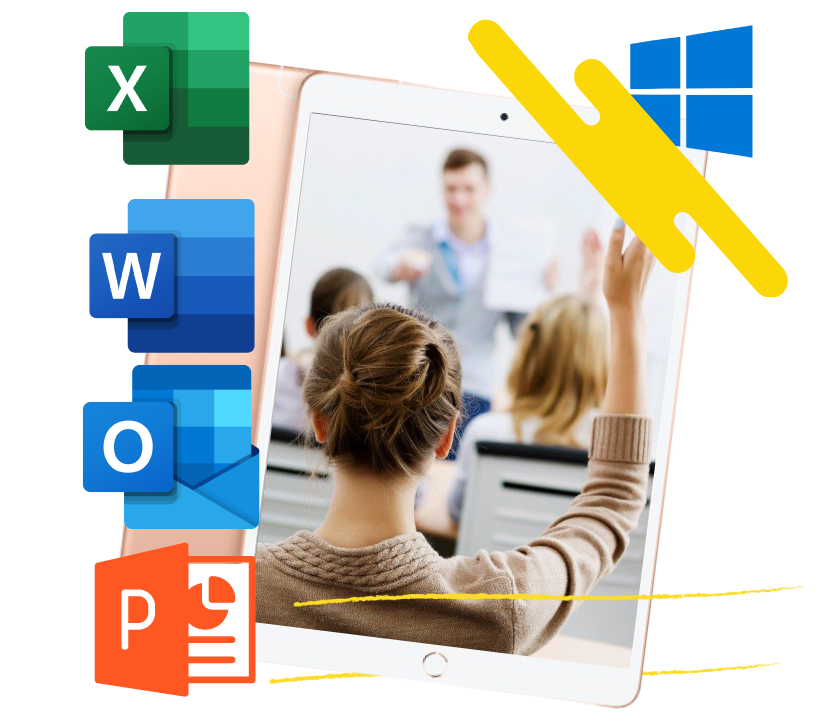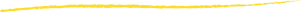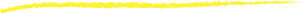# Introduction to DAX for Power BIIn this two-day course, students will be introduced to using Data Analysis Expressions (DAX), which is the expression language that is used to create custom calculations in the Power BI Data model. The course covers some of the theoretical underpinnings of the data model and DAX, but the emphasis is on using DAX to solve common business problems. Students will learn how to write their own calculated columns, measures, and tables, how to visualize the way Power BI computes DAX calculations, and how to troubleshoot custom code. This instructor-led course consists of a combination of lecture, demonstrations, and hands-on labs.

### 2022 Class Schedules

2 day class\$250/ Student